# Test Prep Plan - Take a practice test

Take this practice test to check your existing knowledge of the course material. We'll review your answers and create a Test Prep Plan for you based on your results.
How Test Prep Plans work
1
2Based on your results, we'll create a customized Test Prep Plan just for you!
3Study smarter
Study more effectively: skip concepts you already know and focus on what you still need to learn.

# Limits: Homework Help Chapter Exam

Exam Instructions:

Choose your answers to the questions and click 'Next' to see the next set of questions. You can skip questions if you would like and come back to them later with the yellow "Go To First Skipped Question" button. When you have completed the practice exam, a green submit button will appear. Click it to see your results. Good luck!

### Page 1

#### Question 1 1. The expression below can also be written as: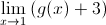#### Question 2 2. Calculate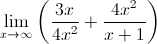#### Question 3 3. Using the graph, find the value of z, then add 1.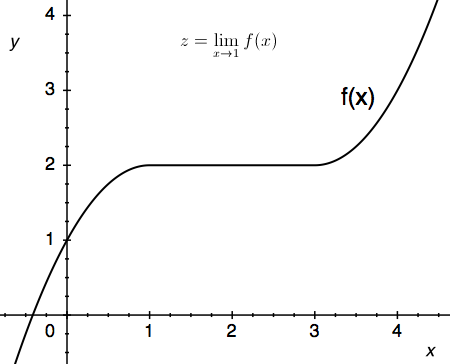#### Question 4 4. Solve for the limit: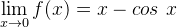#### Question 5 5. Using the given data, solve for the continuous function y.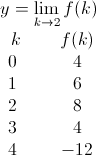### Page 2

#### Question 7 7. The ceiling function is graphically translated as a stepwise function. What is the limit of f(x) = ceil(x) as x approaches 4.5?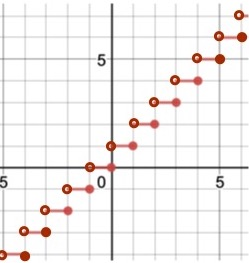#### Question 8 8. Which of the following is true?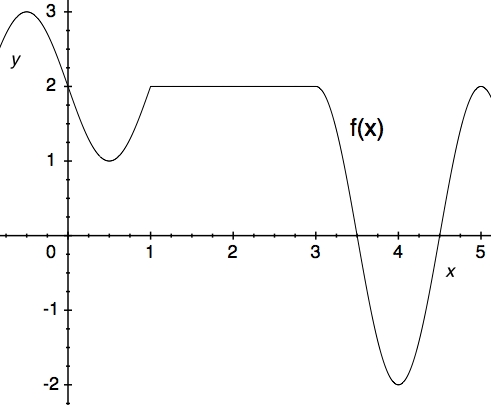### Page 3

#### Question 11 11. Using the squeeze theorem, what is z?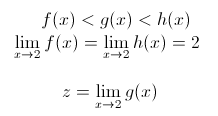#### Question 12 12. Using the squeeze theorem, what is z?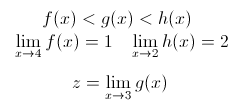#### Question 13 13. Using the squeeze theorem, what is z?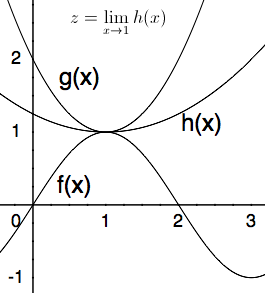#### Question 14 14. The expression below can also be written as: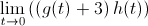#### Question 15 15. Use the graph to solve for z.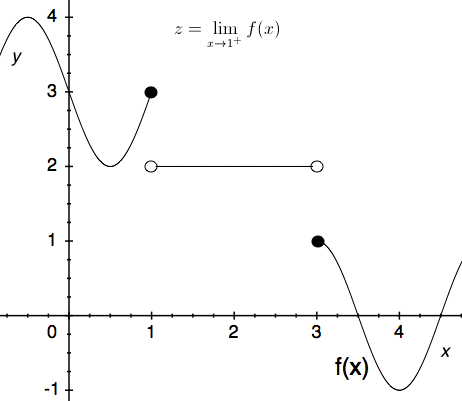### Page 4

#### Question 17 17. Using the squeeze theorem, what is z?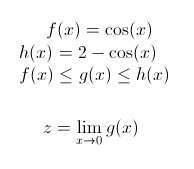#### Question 18 18. Using the squeeze theorem, what is z?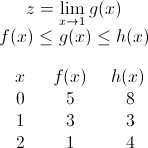#### Question 19 19. The floor function is graphically represented as a stepwise function. What is the limit of f(x) = floor(x) as x approaches 4?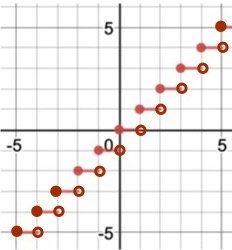#### Question 20 20. Using the given data, predict the likely value of the continuous function z.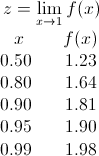### Page 5

#### Question 21 21. Calculate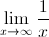#### Question 22 22. Calculate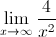#### Question 23 23. Calculate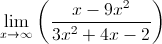#### Question 24 24. Calculate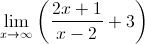### Page 6

#### Question 26 26. Use the given formula to solve for z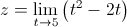#### Question 27 27. Use the graph to solve for z.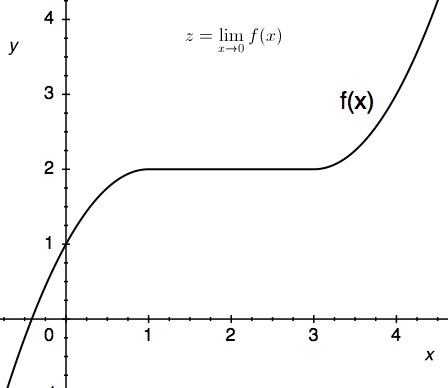#### Question 28 28. Evaluate the limit below.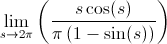#### Question 29 29. Use the graph to solve for z.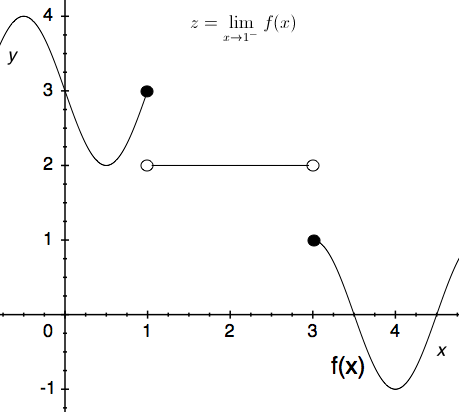#### Limits: Homework Help Chapter Exam Instructions

Choose your answers to the questions and click 'Next' to see the next set of questions. You can skip questions if you would like and come back to them later with the yellow "Go To First Skipped Question" button. When you have completed the practice exam, a green submit button will appear. Click it to see your results. Good luck!

Support